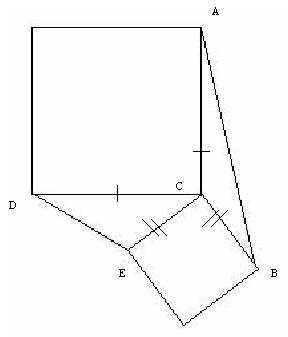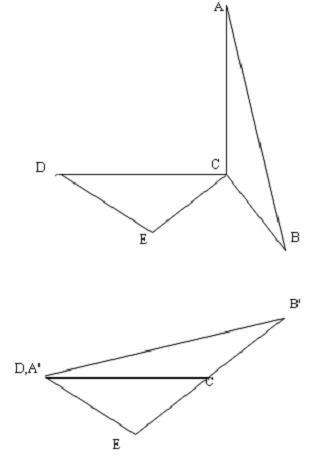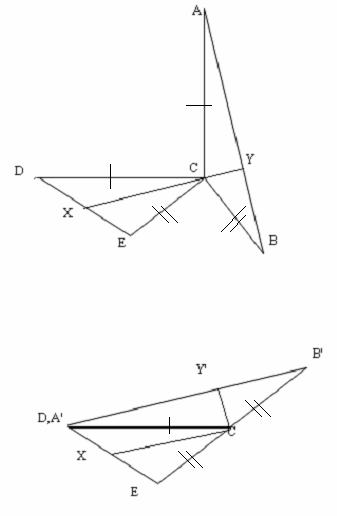#### You may also like### Fixing It

A and B are two fixed points on a circle and RS is a variable diamater. What is the locus of the intersection P of AR and BS?### OK! Now Prove It

Make a conjecture about the sum of the squares of the odd positive integers. Can you prove it?### Summats Clear

Find the sum, f(n), of the first n terms of the sequence: 0, 1, 1, 2, 2, 3, 3........p, p, p +1, p + 1,..... Prove that f(a + b) - f(a - b) = ab.

# Trig Rules OK

##### Age 16 to 18 Challenge Level:Curt from Reigate College has a neat way of proving this result by rotating one of the triangles about $C$, keeping the other one fixed, and then using similar triangles. It is illuminating to see different methods and you may also like to try proving this result using the Sine Rule. Here is Curt's method. The question specifies the construction of a line perpendicular to $AB$ drawn through $C$, and continued until it intersects $DE$. It will be proven that the perpendicular to $AB$ through $C$ bisects $DE$. To prove this we first develop a useful tool. Note that $\angle BCE=\pi/2$, thus if we were to rotate $B$ by $\pi/2$ radians counter-clockwise to $B'$, then ECB' would be a straight line. Also we note that $\angle ACD= \pi/2$. If we were to rotate $A$ by $\pi/2$ counter clockwise to $A'$, then $A'$ would coincide with $D$ as $|CD |=|CA|=|CA'|$.The diagram shows the results of rotating triangle $ABC$ by $\pi/2$ counter clockwise. As lengths between points are invariant under such rotations, $|CA| = |CA'|$, $|AB|=|A'B'|$ and $|BC| =|B'C|$ thus $A'B'C$ is the same triangle as $ABC$. Clearly $EDC$ is unaffected by the rotation; none of its vertices were rotated or translated. Now in order to see how this is useful, we start from the first diagram, and draw in the extended perpendicular from $Y$ on $AB$ passing through $C$ intersecting $DE$ at $X$. We repeat the rotation about $C$ as shown in the next diagram.In addition to the other transformations already discussed, $Y$ goes to $Y'$. $XCY$ is a straight line perpendicular to $AB$, and $Y$ is rotated $\pi/2$ about $C$ to $Y'$. Thus $XCY'$ is a right angle and $A'B'$ is parallel to $XC$. Again, as the distances between points that are rotated in the same manner are invariant under rotation, it follows that $|B'Y'|=|BY|$ and $|A'Y'| = |AY|$. It is out intention to show that $|DX| = |EX|$, or $|DE| = 2|DX|$
Clearly $ECX$ is similar to $EBD'$.

$CX$ is parallel to $DB'$ thus $\angle EB'D = \angle ECX$. Similarly angle $\angle EXC = \angle EDB'$. Both triangles share $\angle DEB'$. Thus all three angles in the two triangles are equal, thus the two triangles are similar.

By the properties of similar triangles, $|EB'|/|EC| = |ED|/|EX|$. But $|EB'| = 2|EC|$. Thus $ED = 2|EX|$, as required.